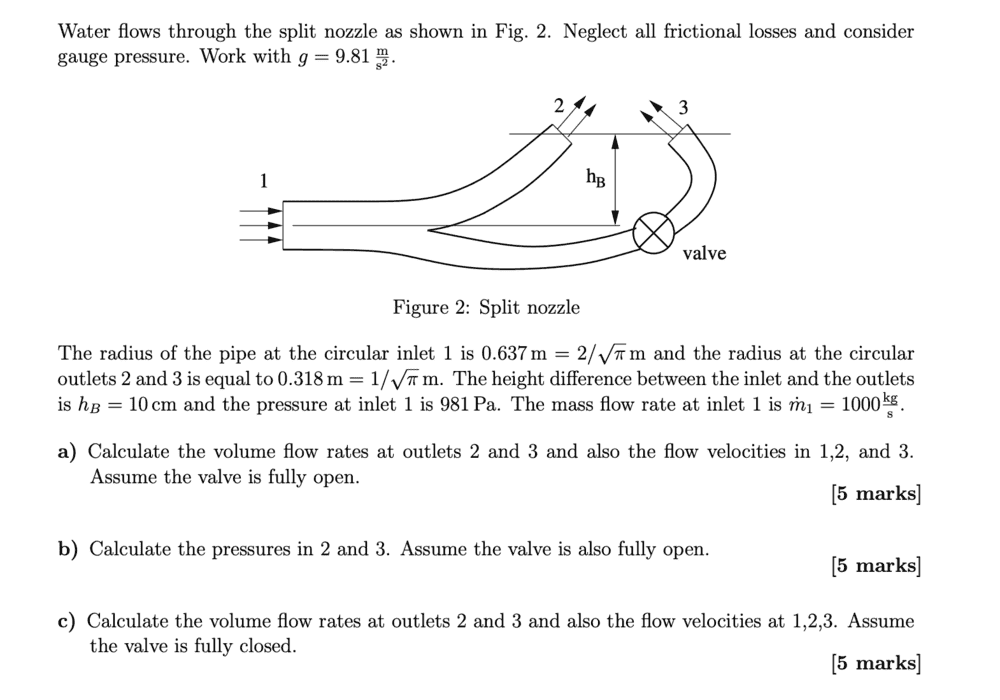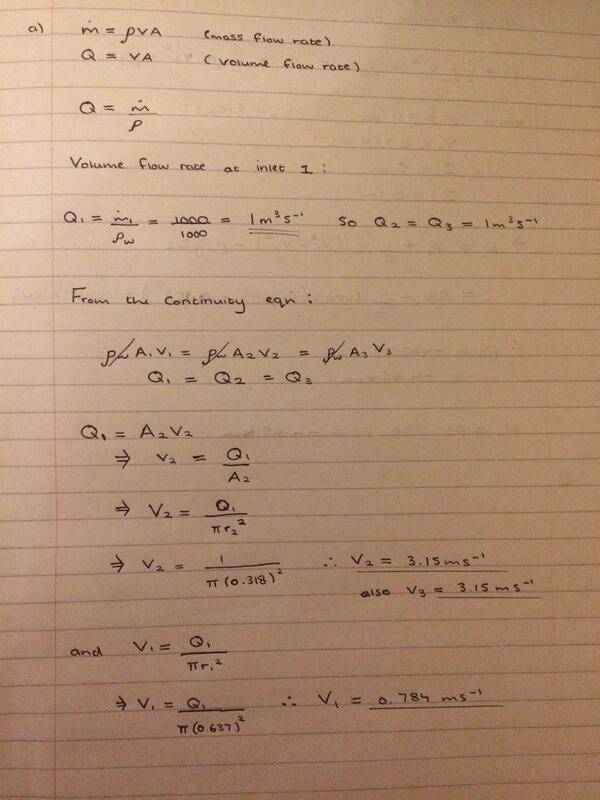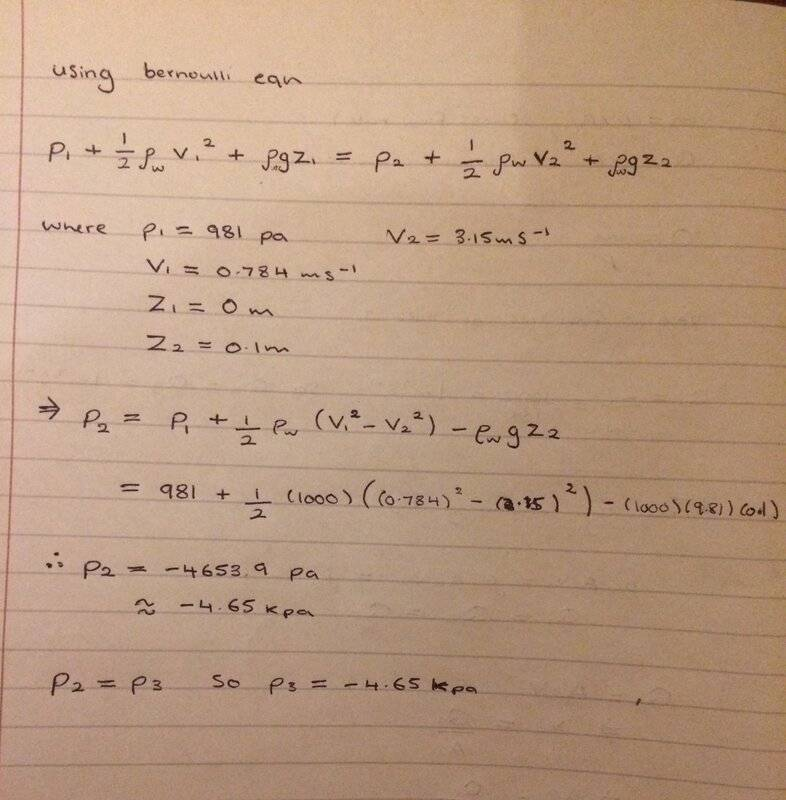# Volume flow rate

• Bolter

#### Bolter

Homework Statement
See below
Relevant Equations
volume flow rate = vA
Made an attempt at this Q but I'm unsure on how to do part c) or if I had even done part a) and b) rightWhat difference does it make to the volume flow rate equation when the valve goes from open to closed?

Any help would be appreciated! Thanks

How can there be 1m3/s flowing in and 1m3/s going out of both outlets?

How can there be 1m3/s flowing in and 1m3/s going out of both outlets?

So do I assume that the inlet has 1 m^3/s flowing in
and both outlets at the end have 0.5 m^3/s flowing out

So do I assume that the inlet has 1 m^3/s flowing in
and both outlets at the end have 0.5 m^3/s flowing out
For this system, yes.